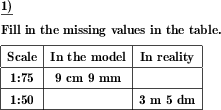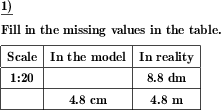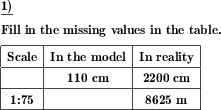Custom math worksheets at your fingertips# Details for problem "Complete the table with scaled lengths"

Quickname: 3223

Elementary School, Primary School, Junior High School, Middle School.

## Summary

In a table with scale, scaled length and real length, missing values must be added

## Examples## Description

Scales are used on maps to measure reality distances. In a table showing scale, displayed length, and real length, missing values must be added.

In this worksheet, conversion has to be done for missing values based on the other two given values.

This versatile worksheet is perfect for group activity. Teamwork and discussion on the topic will enhance the conversion skills. It can also be used for independent learning and homework practice.

Practicing these worksheets will build up the following skills.

1. Apply multiplication and division concepts while converting the values.
2. Revise the concept of reading scales according to set values.
3. In-depth understanding of conversion between scale, in the model measurement, and based on the real measurement.
4. Reading scales and converting between units of measure with more confidence, will be achieved.
5. Decimal numbers exercise will be done.
6. Grasp the skill to use different units of measurement accordingly.

These worksheets will boost critical thinking and problem-solving skills in the context of real-life problems. The number of problems, scaling factor, direction of conversion, and notation of length specification can be chosen.

• Each task can be generated with the number of problems starting from 1 problem and a maximum of 10 problems per tasks.
• The scaling factor is up to 500000.
• Notation of length specifications can also be selected in the customizable worksheet.

The answer key will provide all missing values in the table.

## Free worksheets and solution sheets with answers for download

Download free printable worksheets for this math problem here. The worksheet contains the problems only, the solution sheet includes the answers. Just click on the respective link.

•Worksheet 1Solution sheet with answers
•Worksheet 2Solution sheet with answers
•Worksheet 3Solution sheet with answers

If you can not see the solution sheets for download, they may be filtered out by an ad blocker that you may have installed. If this is the case, please allow ads for this page and reload the page. The solution sheets will then reappear.

• Do these sample worksheets do not really fit?
• Do you need more math worksheets, with a different level of difficulty?
• Would you like to combine different problems on a worksheet and adjust them to your needs?
• As a teacher, you can put together your own worksheets using the automatically generated math problems provided.
With a free initial credit, you can start creating your own math worksheets in a few minutes.

You can try it for free! Register here, to create custom worksheets now!

## Customization options for this problem

Parameter
Possible values
Number of problems
1, 2, 3, 4, 5, 6, 7, 8, 9, 10
Scaling factor
1:5-1:100, 1:10000-1:500000
Notation of length specification
in multiple unit steps, in decimal notation, whole numbers only

## Similar problems

Remark
Description
Name and direct link
Only the lengths are to be converted in both directions
Conversion of lengths at a given scale in both directions
Only the scale factor has to be determined
The scale used for an illustration shall be determined.You may pass on the worksheets offered for download on this page to others, but not modify or sell them.